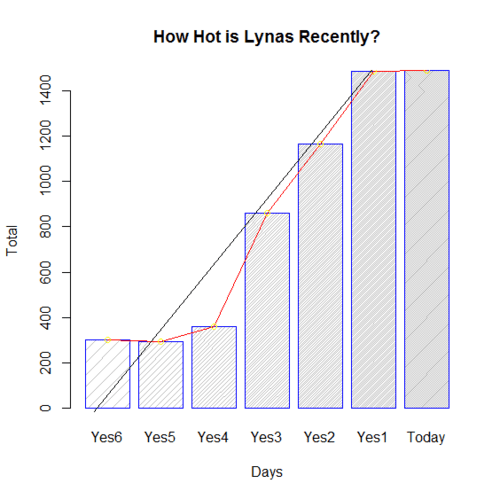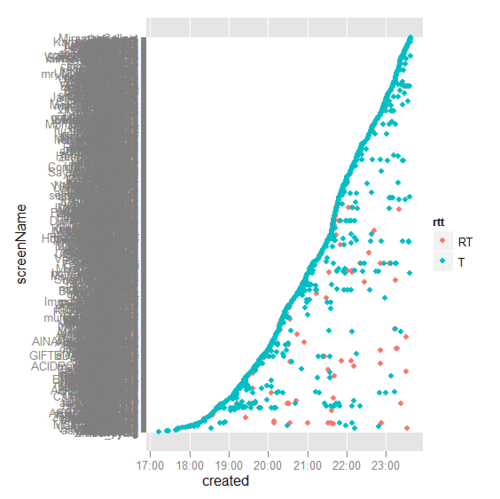Want to share your content on R-bloggers? click here if you have a blog, or here if you don't.

Since Lynas Malaysia Corporation, Australia Rare Earth Refiner Granted Malaysia License, it is always a very hot topic. This can be proven through the daily tweets across recent 7 days. This chart was built by Tweets Keyword Trending Application @ http://www.cloudstat.org/index.php?do=/kaichew/blog/tweets-keyword-trending-application/

Result:> xA
  300  293  359  860 1167 1488 1490Source code:

z=1:7

#Today
x0 <- length(s0) #Yesterday
s1 <- searchTwitter(y, n, since=as.character(Sys.Date()-1), until=as.character(Sys.Date()))
x1 <- length(s1) #Yesterday2
s2 <- searchTwitter(y, n, since=as.character(Sys.Date()-2), until=as.character(Sys.Date()-1))
x2 <- length(s2) #Yesterday3
s3 <- searchTwitter(y, n, since=as.character(Sys.Date()-3), until=as.character(Sys.Date()-2))
x3 <- length(s3) #Yesterday4
s4 <- searchTwitter(y, n, since=as.character(Sys.Date()-4), until=as.character(Sys.Date()-3))
x4 <- length(s4) #Yesterday5
s5 <- searchTwitter(y, n, since=as.character(Sys.Date()-5), until=as.character(Sys.Date()-4))
x5 <- length(s5) #Yesterday6
s6 <- searchTwitter(y, n, since=as.character(Sys.Date()-6), until=as.character(Sys.Date()-5))
x6 <- length(s6) xA <- c(x6, x5, x4, x3, x2, x1, x0) df.bar <- barplot(xA) barplot(xA, main=”How Hot is Lynas Recently?”, xlab=”Days”,
ylab=”Total”, names.arg=c(“Yes6”,”Yes5”,”Yes4”,”Yes3”,”Yes2”,”Yes1”,”Today”),
border=”blue”, density=c(10,20,30,40,50,60,70))
lines(x = df.bar, y = xA, col=”red”)
points(x = df.bar, y = xA, col=”yellow”)
abline(lm(xA~z))
#

Last but not least. Save Malaysia, Stop Lynas!